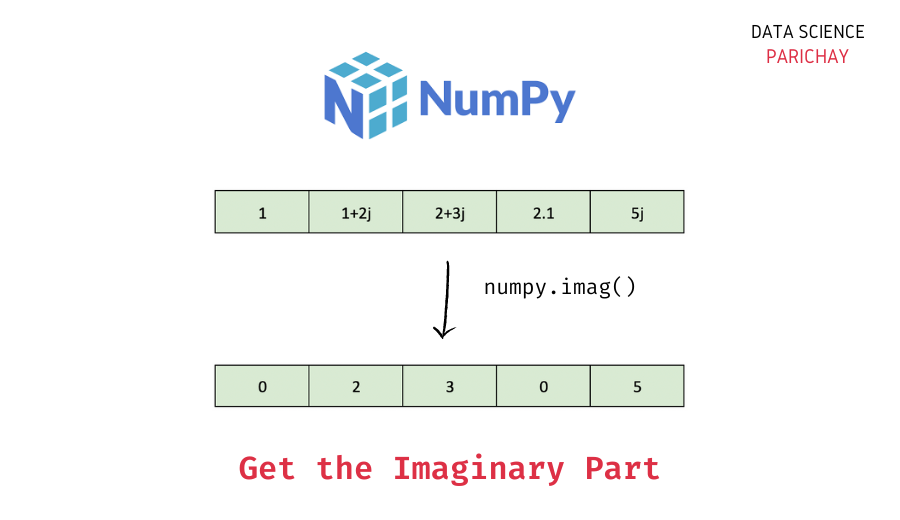# Numpy – Get the Imaginary Part of a Complex Number

In this tutorial, we will look at how to get the imaginary part of a complex number in a Numpy array. For example for the complex number `a + bj`, `a` is the real part and `b` is the imaginary part.

## How to get the imaginary part of complex values in a Numpy array?

You can use the `numpy.imag()` function to get (element-wise) the imaginary part of complex numbers in a Numpy array. The following is the syntax –

```# get the imaginary part - pass scaler value or numpy array
np.imag(a)```

The `numpy.imag()` function essentially returns (element-wise) the imaginary component of the complex argument.

If you pass a scalar value, it returns the imaginary part of that value. And, if you pass a Numpy array, it returns the array of imaginary parts of the array elements.

## Examples

Let’s now look at some examples of using the above function to extract the imaginary part of complex numbers.

### Example 1 – Extract the imaginary part for a scalar value using `numpy.imag()`

First, let’s pass scaler values to the `numpy.imag()` function.

Let’s apply the `numpy.imag()` function on three scalar values – a real number, a complex number with a non-zero real part, and a complex number with the real part as 0.

```import numpy as np

# get the imaginary part
print(np.imag(14))
print(np.imag(2 + 4j))
print(np.imag(3j))```

Output:

📚 Data Science Programs By Skill Level

Introductory

Intermediate ⭐⭐⭐

🔎 Find Data Science Programs 👨‍💻 111,889 already enrolled

Disclaimer: Data Science Parichay is reader supported. When you purchase a course through a link on this site, we may earn a small commission at no additional cost to you. Earned commissions help support this website and its team of writers.

```0
4.0
3.0```

We get the imaginary component for all the scalar values irrespective of whether we passed a real or a complex number as an argument.

### Example 2 – Element-wise extract the imaginary part in a Numpy array using `numpy.imag()`

If you apply the `numpy.imag()` function on an array, it will return a Numpy array of the extracted imaginary components for the elements inside the array.

Let’s create a 1-D array and apply the `numpy.imag()` function to it.

```# create a numpy array
ar = np.array([1, 2+3j, 2+0j, 0+4j, 5.7, np.nan, np.inf, 1j, 0+0j])
# element-wise extract the imaginary part in ar
np.imag(ar)```

Output:

`array([0., 3., 0., 4., 0., 0., 0., 1., 0.])`

We get a Numpy array containing the imaginary component of each value in the array `ar`.

## Summary – Imaginary part of complex numbers in Numpy array

In this tutorial, we looked at how we can use the `numpy.imag()` function to extract the imaginary component of a complex number in a Numpy array. Keep in mind that if you apply this function on a scalar value, it returns the imaginary component whereas if you apply it on a Numpy array, it returns the array of imaginary components.

You might also be interested in –

•